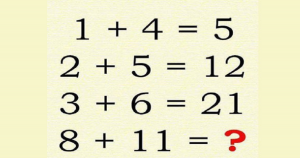# Logic Puzzle With Two Answers Has The Internet Confused. Can You Solve It?

Puzzles are often devised as a form of entertainment but they can also arise from serious mathematical or logistical problems. In such cases, their solution may be a significant contribution to mathematical research.

Recently, a puzzle is going viral on the Internet which is confusing everyone’s minds.

This bizarre puzzle asks you to find a pattern between four equations and it has two answers. Eventually, it led to numerous heated debate online. According to a report, only one out in a 1,000 people can figure out the second answer.

### Check Out the two answers here:

And the two answers to the puzzle are 40 and 96.

Solution-1:

Step-1: Here, you begin with 1 + 4 to get 5.

Step-2: Then, you add 2 + 5 to the first answer of 5 to reach 12.

Step-3: Similarly, adding 3 + 6 to 12 gives 21.

Step-4: In the end, 8 + 11 + 21 = 40.

The quick answer, therefore, is 40, arrived at by simply adding each equation with the sum from the previous equation.

Solution-2:

It is a bit complicated.

Step-1: One way to arrive at the answer of 5 in the first equation is to add 1 to (4 x 1)

Step-2: Following that rule, other equations will be solved like this:

2 + (2 x 5) = 12

3 + (3 x 6) = 21

8 + (8 x 11) = 96

Though the puzzle looks simple, many of the people could get only one solution of either. The puzzle has received some hate on the Internet too. However, many have pointed out that both the answers are correct since it is not a math puzzle but a logic puzzle.

Do you have any such logical puzzles? Share with us in the comments section below.

×